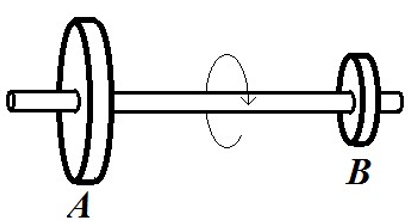Problem: Two solid discs are rotating about a perpendicular shaft through their centers, as shown in the figure. Disc A, has a radius that is twice as large as disc  B,? Which of the following statements is NOT true?A) A point on the rim of disc  A, has twice the linear speed as a point on the rim of disc  B.B) The direction of the angular velocity is to the right.C) Every point on the body has the same angular acceleration.D) The linear acceleration of a point on the rim of disc  B is the same as the linear acceleration of a point halfway from the center to the rim on disc  A.E) The angular velocity at a point on the rim of disc  A is twice the angular velocity of a point on the rim of disc B.

FREE Expert Solution
83% (364 ratings)
Problem Details

Two solid discs are rotating about a perpendicular shaft through their centers, as shown in the figure. Disc A, has a radius that is twice as large as disc  B,? Which of the following statements is NOT true?

A) A point on the rim of disc  A, has twice the linear speed as a point on the rim of disc  B.

B) The direction of the angular velocity is to the right.

C) Every point on the body has the same angular acceleration.

D) The linear acceleration of a point on the rim of disc  B is the same as the linear acceleration of a point halfway from the center to the rim on disc  A.

E) The angular velocity at a point on the rim of disc  A is twice the angular velocity of a point on the rim of disc B.